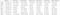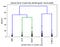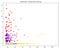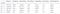Unsupervised Learning With Python — K- Means and Hierarchical Clustering

Machine Learning can be broadly classified into 2 types:

• Supervised Learning — Where a response variable Y is present. Here there could be 2 goals, 1. Find f(X)=Y, such that f(X) closely approximates Y or 2. Predicting the value of Y given X.Usually, Regression, Decision trees, Random Forest, SVM, Naive Bayes etc.are used for these kind of problems
• Unsupervised Learning — Where there is no response variable Y and the aim is to identify the clusters with in the data based on similarity with in the cluster members. Different algorithms like K-means, Hierarchical, PCA,Spectral Clustering, DBSCAN Clustering etc. are used for these problems

In real life, the unsupervised learning is more useful, as this data is available easily and is less expensive — as its mostly machine generated data. Data with response variable is expensive because it requires some human intervention to tag the observations as belonging to certain class or identifying the outputs

In this article, the aim is to apply the K-means and Hierarchical clustering to AirlinesCluster dataset on Kaggle. For indepth understanding of how the clustering algorithms function , please refer to excellent resources online like the Introduction to Statistical Learning with R book and video lectures by Gareth James, Daniela Witten, Trevor Hastie and Robert Tibshirani. The link for the book — ISLR

PATH = "../input"
#importing the dataset
#creating a duplicate dataset to work on
dataset1 = dataset
# peeking at the dataset
#Descriptive stats of the variables in data
dataset1.describe()Descriptive Statistics of the Airline Cluster data

Standardizing the dataset is essential , as the K-means and Hierarchical clustering depend on calculating distances between the observations. Due to different scales of measurement of variables, some variables may have higher influence on the clustering output

#standardize the data to normal distribution
from sklearn import preprocessing
dataset1_standardized = preprocessing.scale(dataset1)
dataset1_standardized = pd.DataFrame(dataset1_standardized)

In K-means, the number of clusters required has to be decided before the application, so some level of domain expertise would of help. Else we can use a scree plot to decide number of clusters based on reduction in variance

# find the appropriate cluster number
plt.figure(figsize=(10, 8))
from sklearn.cluster import KMeans
wcss = []
for i in range(1, 11):
kmeans = KMeans(n_clusters = i, init = 'k-means++', random_state = 42)
kmeans.fit(dataset1_standardized)
wcss.append(kmeans.inertia_)
plt.plot(range(1, 11), wcss)
plt.title('The Elbow Method')
plt.xlabel('Number of clusters')
plt.ylabel('WCSS')
plt.show()

K-Means Clustering

# Fitting K-Means to the dataset
kmeans = KMeans(n_clusters = 5, init = 'k-means++', random_state = 42)
y_kmeans = kmeans.fit_predict(dataset1_standardized)
#beginning of the cluster numbering with 1 instead of 0
y_kmeans1=y_kmeans
y_kmeans1=y_kmeans+1
# New Dataframe called cluster
cluster = pd.DataFrame(y_kmeans1)
# Adding cluster to the Dataset1
dataset1['cluster'] = cluster
#Mean of clusters
kmeans_mean_cluster = pd.DataFrame(round(dataset1.groupby('cluster').mean(),1))
kmeans_mean_cluster

Hierarchical Clustering

# Hierarchical clustering for the same dataset
# creating a dataset for hierarchical clustering
dataset2_standardized = dataset1_standardized
# needed imports
from matplotlib import pyplot as plt
from scipy.cluster.hierarchy import dendrogram, linkage
import numpy as np
# some setting for this notebook to actually show the graphs inline
# you probably won't need this
%matplotlib inline
np.set_printoptions(precision=5, suppress=True) # suppress scientific float notation
#creating the linkage matrix
plt.title('Hierarchical Clustering Dendrogram (truncated)')
plt.xlabel('sample index or (cluster size)')
plt.ylabel('distance')
dendrogram(
H_cluster,
truncate_mode='lastp', # show only the last p merged clusters
p=5, # show only the last p merged clusters
leaf_rotation=90.,
leaf_font_size=12.,
show_contracted=True, # to get a distribution impression in truncated branches
)
plt.show()Dendogram — Hierarchical Clustering of Airline Customer
# Assigning the clusters and plotting the observations as per hierarchical clusteringfrom scipy.cluster.hierarchy import fcluster
k=5
cluster_2 = fcluster(H_cluster, k, criterion='maxclust')
cluster_2[0:30:,]
plt.figure(figsize=(10, 8))
plt.scatter(dataset2_standardized.iloc[:,0], dataset2_standardized.iloc[:,1],c=cluster_2, cmap='prism') # plot points with cluster dependent colors
plt.title('Airline Data - Hierarchical Clutering')
plt.show()Airline Customer Cluster — Hierarchical Clustering

Adding the assigned hierarchical clusters data to the dataframe and calculating the means of the features of the clusters

# New Dataframe called cluster
cluster_Hierarchical = pd.DataFrame(cluster_2)
# Adding the hierarchical clustering to dataset
dataset2=dataset1
dataset2['cluster'] = cluster_HierarchicalHierarchical Clustering — Means of features

Insights and Plan of Action:

1. Cluster 5 is set of the recently acquired customer group as the Days since enrollment is lowest , moreover their flight transactions in last 12 months as well as the qualified miles for top class travel is the lowest.
2. Cluster 3 is the set of high vintage customers who have highest number of non-flight bonus transaction miles and highest miles eligible for award travel
3. Cluster 4 is also high vintage customers however their number of flight miles and flight transactions in last 12 months is alarmingly low, they may churn unless some intervention is done. Bespoke offers to activate these customers is necessary
4. Cluster 2 is group of customers who have done highest number of flight transactions and acquired flight miles in last 12 months. Investigate further and identify their needs. For Eg: They may be baby boomers generation who have begun to travel around after their retirement etc.

Related Posts from DDI:

Data Driven Investor

from confusion to clarity, not insanity

Welcome to a place where words matter. On Medium, smart voices and original ideas take center stage - with no ads in sight. Watch
Follow all the topics you care about, and we’ll deliver the best stories for you to your homepage and inbox. Explore
Get unlimited access to the best stories on Medium — and support writers while you’re at it. Just \$5/month. Upgrade